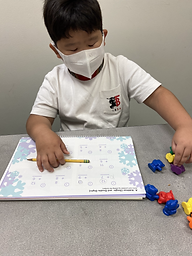Ms. Joan

Target 1​

Continuation

Number Operation

:

Computation

1:

Recognize the arithmetic symbols in an addition equation: plus (+) and equals (=).

2:

Understand that addition means to put two quantities together.

3:

Understand that adding numbers means making a larger number.

4:

Understand that addition equations can be written both vertically and horizontally.

Kindergarden

Vocabulary:

Activities:

Students were given random dominoes and use that to add.

• Students were given 2 digit and 1-digit number to add on the board writing it horizontally and vertically.
• Using a number line, students explored counting forward as their means to find the sum starting from the biggest number.
• Students solve given problem by identifying the number with the highest value and count forward using counting bears.Home Exploration

Guiding Questions:Absent Students:

Target 2

:

Vocabulary:

Activities:Home Exploration

Guiding Questions:Target 3

:

Vocabulary:

Activities:Home Exploration